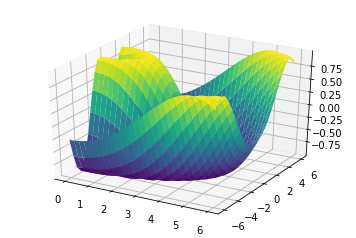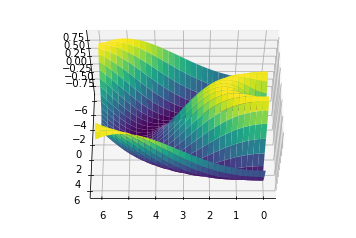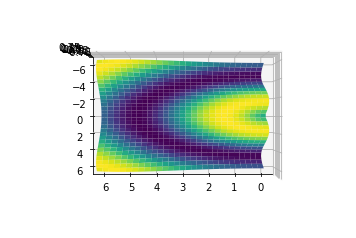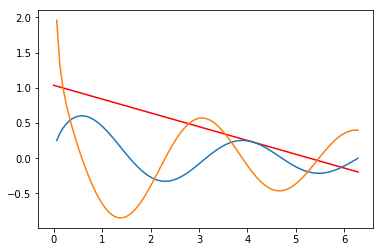Surya Dantuluri's Blog

# Gradients, Backprop, and Derivatives in Tensorflow [Draft]

## Tutorial on computing gradients and derivatives using Numpy and Tensorflow

$$f(x,y) = \cos{x}*\sin{y} + \frac{x}{y}$$

## Using Numpy, Autograd, and Tensorflow¶

In :
#Dependencies
import tensorflow as tf
from mpl_toolkits import mplot3d
import numpy as np
import matplotlib.pyplot as plt
%matplotlib inline

In :
import autograd.numpy as np  # Thinly wrapped version of numpy

def f(x, y):
return np.sin(np.sqrt(x ** 2 + y ** 2))

x = 2.
y = 0.

print (f(x, y))

0.9092974268256817
-0.4161468365471424

In :
# Graph

def f(x, y):
return np.sin(np.sqrt(x ** 2 + y ** 2))

x = np.linspace(0, 6, 30)
y = np.linspace(-6, 6, 30)

X, Y = np.meshgrid(x, y)

Z = f(X, Y)
fig = plt.figure()
ax = plt.axes(projection='3d')
ax.contour3D(X, Y, Z, 50, cmap='binary')
# ax.set_xlabel('x')
# ax.set_ylabel('y')
# ax.set_zlabel('z')
ax.set_xlabel('X axis')
ax.set_ylabel('Y axis')
ax.set_zlabel('Z axis')

ax = plt.axes(projection='3d')
ax.plot_surface(X, Y, Z, rstride=1, cstride=1,cmap='viridis',edgecolor='none')

Out:
<mpl_toolkits.mplot3d.art3d.Poly3DCollection at 0xb1f8b4cc0>In :
ax.view_init(60, 90)
fig

Out:In :
ax.view_init(90, 90)
fig

Out:In [ ]:
# Computing gradients with some Numpy

# Method 1: Using Partial Derivatives

# def f(x, y):
#     return np.cos(x)*np.sin(y)+(x/y)

# # Compute f(x,y) = cos(x)*sin(x)+(x/y)
# x = input()
# y = input()

# # Forward pass
# w5 = np.cos(x)*np.sin(y)+(x/y) # Node 1
# w6 = (x/y)                     # Node 2

# # Backward pass
# dw7_d
# df_dq = z          # Node 2 input
# df_dz = q          # Node 2 input
# df_dx = 2 * df_dq  # Node 1 input
# df_dy = 1 * df_dq  # Node 1 input

# grad = np.array([df_dx, df_dy, df_dz])

# print f


# Using tf.gradient to find derivatives¶

In :
%matplotlib inline
import tensorflow as tf
import numpy as np
from math import pi
import matplotlib.pyplot as mp

tf.reset_default_graph()

# Length of graph
length = np.linspace(0,pi*2,100)

# Point at which we want to find derivative at
x = tf.placeholder(tf.float32)

# Function
y=(1/tf.sqrt(x))*(tf.cos(x)*tf.sin(x))

# Running tf session
with tf.Session() as session:
dy  = session.run(y,feed_dict={x:length})
print("Enter input number")
input_number = float(input())
print("Done inputting number")
y_at = (1/np.sqrt(input_number))*(np.cos(input_number)*np.sin(input_number))
x_at = input_number
print(y_at)
print(x_at)
function = grad_at*(length - x_at) + y_at
writer = tf.summary.FileWriter('logs', session.graph)
writer.close()

mp.plot(length, function, '-r')
mp.plot(length,dy)

Enter input number
4.1
Done inputting number
[-0.19582547]
0.23229685332631406
4.1

Out:
[<matplotlib.lines.Line2D at 0xb23471a20>]## Graph of f(x), F(x), and tan line to f(x) at inputted point¶

### Red line is f(x) at indicated point¶# Tensorboard of this computational graph¶

One thing to point out: there are two gradients being computed. This is because I computed the gradient twice for graphing and for the tan line. Obviously I could fix this, but the code could be easier for some to understand if the gradient is computed twice.

writes articles on Machine Learning, Full Stack Development, and Insightful Topics